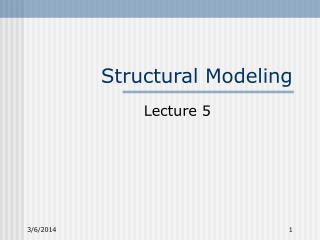# Structural Modeling - PowerPoint PPT PresentationDownload PresentationStructural Modeling

Structural Modeling
Download Presentation## Structural Modeling

- - - - - - - - - - - - - - - - - - - - - - - - - - - E N D - - - - - - - - - - - - - - - - - - - - - - - - - - -
##### Presentation Transcript

1. Structural Modeling Lecture 5

2. Set of Logic Values • Sometimes, {0, 1} is not enough a If a = b = 0, c = ? 0 c If a = b = 1, c = ? 1 b ELEN 468

3. Logic Values in Verilog • 0: Logical 0 • 1: Logical 1 • X: unknown • Z: high impedance ELEN 468

4. X and Z a • a = b = 0: c = Z • a = b = 1: c = X • Initially, every line is X • X is used in simulation. In real circuit, the value is determined by the circuit 0 c 1 b ELEN 468

5. X/X-bar Problem X a = ? • b = X-bar • But because in Verilog, there is no such thing as “X-bar” • b = X, and therefore a = X, which should be 0 b ELEN 468

6. Verilog Nets • wire (default) • tri • wand • wor • triand • trior tri triand/trior wand/wor Not recommended! ELEN 468

7. Example ctrl tri y; bufif1(y, x, ctrl); triand y; bufif1(y, x1, ctrl1); bufif1(y, x2, ctrl2); y x ctrl1 x1 ctrl2 y x2 ELEN 468

8. Truth Tables wire/tri 0 1 x z 0 0 x x 0 1 x 1 x 1 x x x x x z 0 1 x z triad / wand 0 1 x z 0 0 0 0 0 1 0 1 x 1 x 0 x x x z 0 1 x z trior/wor 0 1 x z 0 0 1 x 0 1 1 1 1 1 x x 1 x x z 0 1 x z ELEN 468

9. More Verilog Nets Vdd tri0 • supply0 • supply1 • tri0 • tri1 • trireg Vdd supply1 supply0 Gnd Gnd tri1 a b when a = b = 0, the line maintains its value trireg ELEN 468

10. Signal Strength • 0 and 1 are strong values • supply0 and supply1 provide strong 0 and 1 • tri0 and tri1 provide weak 0 and 1 • Z has no value so it can be overwritten by any of above ELEN 468

11. Registers • reg: store logic value • integer: support computation, loops • real: store delay values • time: store time as 64-bit value • realtime: store time as real number reg one_bit; reg [31:0] one_word; int i; ELEN 468

12. Ports • Net variable: input, output, inout • Register variable: output module B(b,…); inout b; … module A; reg a; B Inst(a,…); module A(a, …); input a; reg a; ELEN 468

13. Memory • Memory is a collection of registers • reg [31:0] cache [0:1023]; • 1 k memory of 32-bit words • reg [31:0] one_word; • reg [7:0] one_byte; • Reference can be made to only a word • one_word = cache; • one_byte = word; ELEN 468

14. Scope of a Variable • The scope of a variable defined in a module is within the module. ELEN 468

15. De-Reference • To reference a variable defined inside an instantiated module • X.w • X.Y.Z.w Module A - Instance X wire w Module B - Instance Y Module C - Instance Z wire w ELEN 468

16. De-referencing module testbench(); reg [3:0] a, b; wire [3:0] y; adder M1 (y, a, b); initial \$monitor(\$time,,”%”, M1.c); endmodule module adder(y, a, b); … wire c; … endmodule ELEN 468

17. Constants parameter width = 32, depth = 1024; parameter initial_value = 8’b1001_0110; … reg [width-1:0] memory [0:depth-1]; ELEN 468

18. Operators • Arithmetic: +, -, *, /, % • Negative numbers are in 2’s complement • Bitwise: ~, &, |, ^, ~^ • ~(10010) = 01101 • (110) & (011) = 010 • Reduction: &, ~&, |, ~|, ^, ~^ • &(1001010) = 0 • ^(1001010) = 1 ELEN 468